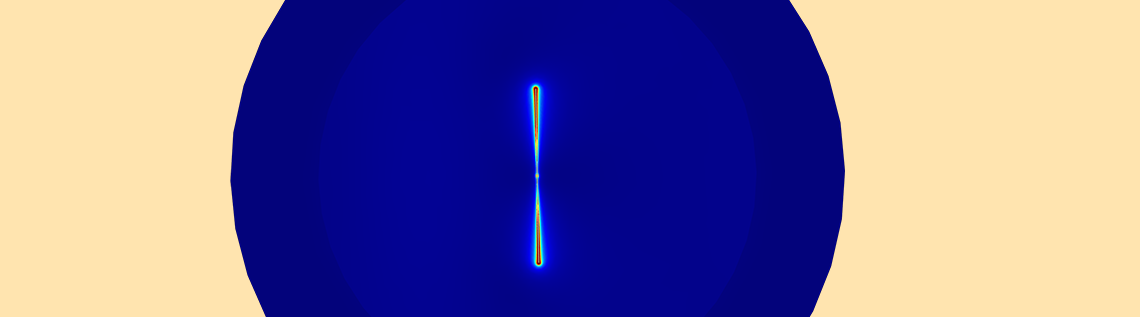# 如何进行全波与射线追踪耦合建模

2017年 1月 30日

### 使用射线光学模块进行多尺度建模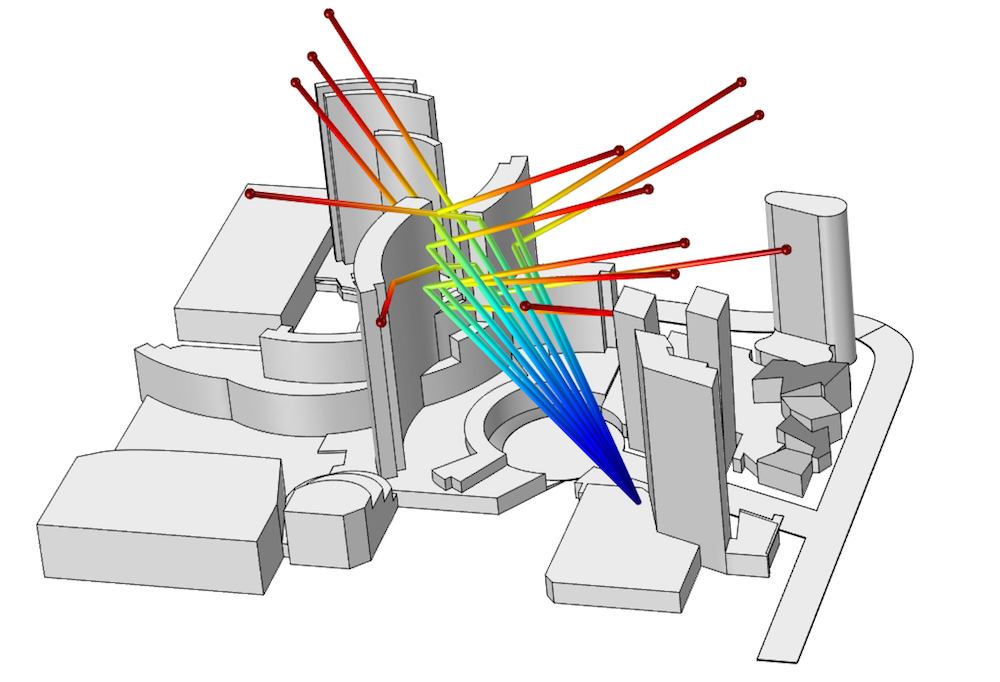Las Vegas（拉斯维加斯）的几家酒店的模型。一个定向天线向 ARIA®Resort＆Casino 辐射射线。

### 天线耦合建模：为射线光学做准备

#### 绘制辐射场

\overrightarrow{E}_{FFx} = emw.Efarx\times \frac{e^{-jkr}}{(r/1[m])}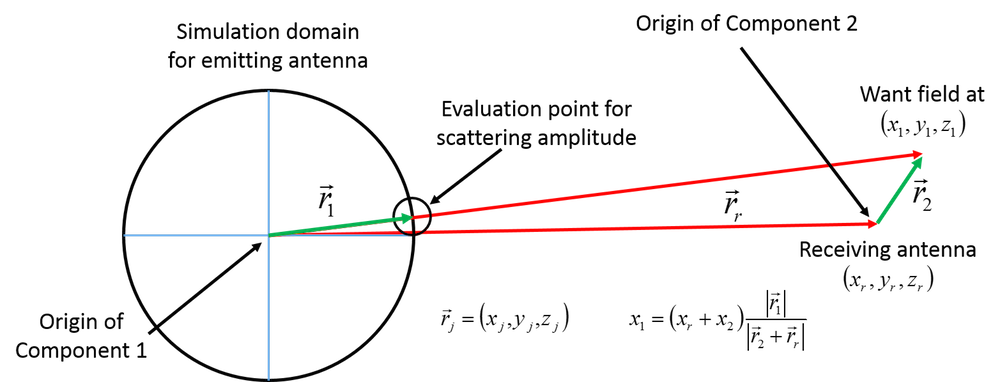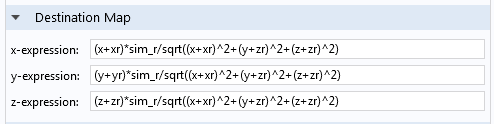#### 在虚拟变量中存储辐射场### 在 COMSOL® 软件中设置几何光学仿真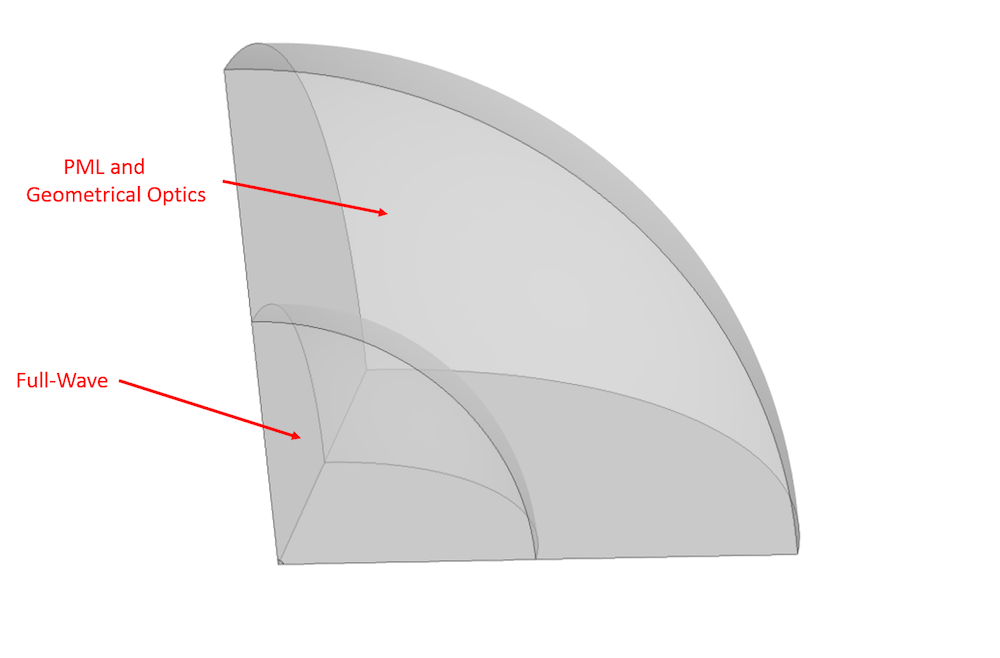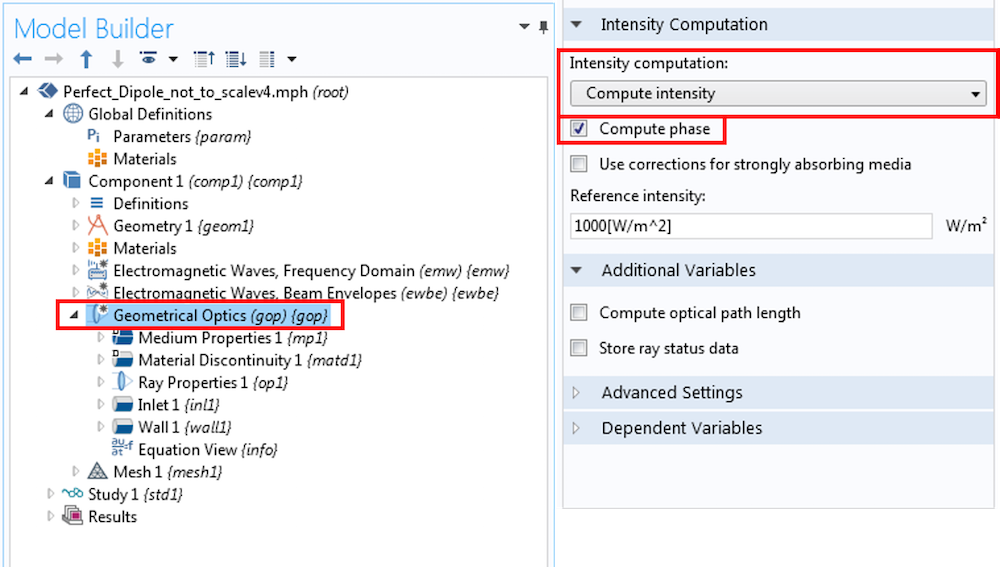Etheta = emw.Efartheta\times \frac{e^{-jkr}}{(r/1[m])}

\begin{align}
\overrightarrow{E} & = \overrightarrow{E}_{FF} + \overrightarrow{E}_{NF} \\
\overrightarrow{E}_{NF} & = \frac{1}{4\pi\epsilon_0}[3\hat{r}(\hat{r}\cdot\vec{p})-\vec{p}](\frac{1}{r^3}+\frac{jk}{r^2})e^{-jkr}\\
\overrightarrow{E}_{FF} & = \frac{1}{4\pi\epsilon_0}k^2(\hat{r}\times\vec{p})\times\hat{r}\frac{e^{-jkr}}{r}\\
\end{align}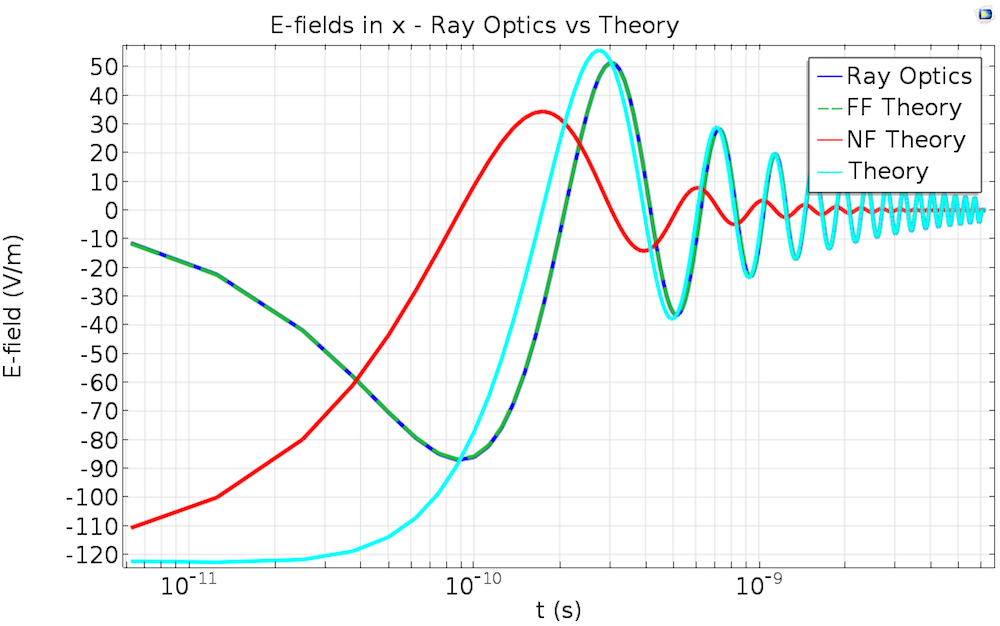### 多尺度建模技术总结

ARIA 是 CityCenter Land，LLC 的注册商标。